# Graph Match

## Match the graphs with their equations or descriptions. A self-marking, drag-and-drop mathematical exercise.

##### GradientLevel 1Level 2Level 3Level 4Exam-StyleDescriptionHelpMore Graph Activities

This is Level 1 (Linear graphs and equations). Match the graphs with the corresponding equations.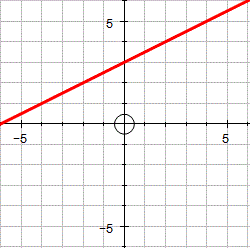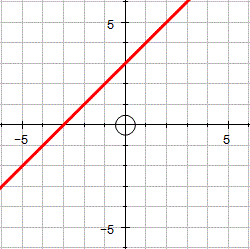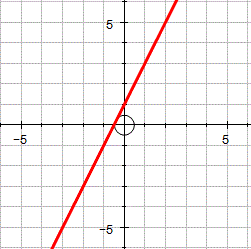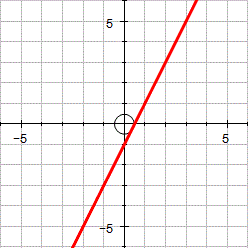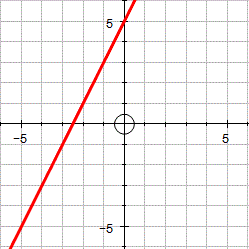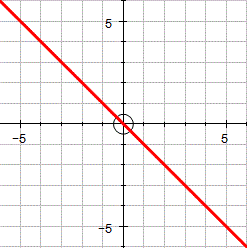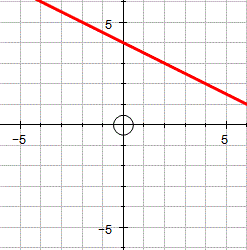$$y = \frac12 x + 3$$

$$y = x + 2$$

$$y = x + 1$$

$$y = x + 3$$

$$y = x$$

$$y = 2x + 1$$

$$y = 2-x$$

$$y = 2x − 1$$

$$y = 2x + 5$$

$$y = - x$$

$$y = x - 1$$

$$y = 4 - \frac12 x$$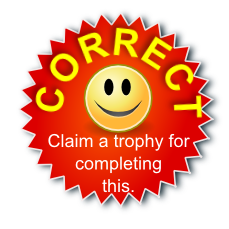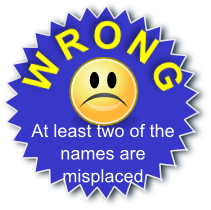The diagrams were created in Autograph.

This is Graph Match level 1. You can also try:
Gradient Level 2 Level 3 Level 4 Exam-style Questions

## Description of Levels

CloseGradient - A pre-requisite for doing the graph exercises is being able to calculate the gradient of a line.

Level 1 - Linear graphs and equations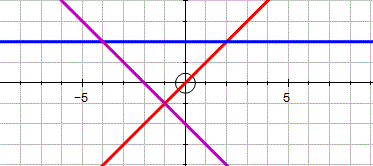Level 2 - Linear and quadratic graphs and equations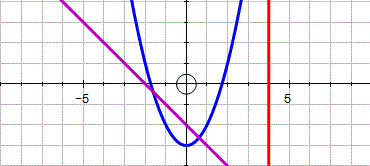Level 3 - Mixed polynomialsLevel 4 - Quadratics in the form $$ax^2 + bx + c$$ given information about the coefficients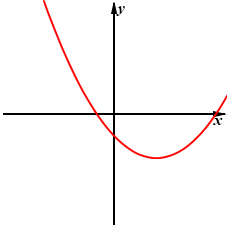Exam Style questions are in the style of GCSE or IB/A-level exam paper questions and worked solutions are available for Transum subscribers.

More on this topic including lesson Starters, visual aids and investigations.

Answers to this exercise are available lower down this page when you are logged in to your Transum account. If you don’t yet have a Transum subscription one can be very quickly set up if you are a teacher, tutor or parent.

For straight line graphs arrange the equation in the form $$y = mx + c$$ where $$m$$ represents the gradient of the line and $$c$$ the y-intercept.

Maybe this video will remind you of some of the techniques for recognising graphs.

This video is from the ukmathsteacher YouTube channel.

Answers to this exercise are available lower down this page when you are logged in to your Transum account. If you don’t yet have a Transum subscription one can be very quickly set up if you are a teacher, tutor or parent.

Close## Transum.org

This web site contains over a thousand free mathematical activities for teachers and pupils. Click here to go to the main page which links to all of the resources available.## More Activities:

Mathematicians are not the people who find Maths easy; they are the people who enjoy how mystifying, puzzling and hard it is. Are you a mathematician?

Comment recorded on the 19 October 'Starter of the Day' page by E Pollard, Huddersfield:

"I used this with my bottom set in year 9. To engage them I used their name and favorite football team (or pop group) instead of the school name. For homework, I asked each student to find a definition for the key words they had been given (once they had fun trying to guess the answer) and they presented their findings to the rest of the class the following day. They felt really special because the key words came from their own personal information."

Comment recorded on the 23 September 'Starter of the Day' page by Judy, Chatsmore CHS:

"This triangle starter is excellent. I have used it with all of my ks3 and ks4 classes and they are all totally focused when counting the triangles."

#### Without Lifting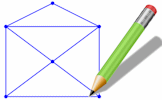Can you draw these diagrams without lifting your pencil from the paper? This is an interactive version of the traditional puzzle. Some diagrams are possible while others are not. What is the rule?

There are answers to this exercise but they are available in this space to teachers, tutors and parents who have logged in to their Transum subscription on this computer.

A Transum subscription unlocks the answers to the online exercises, quizzes and puzzles. It also provides the teacher with access to quality external links on each of the Transum Topic pages and the facility to add to the collection themselves.

Subscribers can manage class lists, lesson plans and assessment data in the Class Admin application and have access to reports of the Transum Trophies earned by class members.

Subscribe

## Go Maths

Learning and understanding Mathematics, at every level, requires learner engagement. Mathematics is not a spectator sport. Sometimes traditional teaching fails to actively involve students. One way to address the problem is through the use of interactive activities and this web site provides many of those. The Go Maths main page links to more activities designed for students in upper Secondary/High school.

## Teachers

If you found this activity useful don't forget to record it in your scheme of work or learning management system. The short URL, ready to be copied and pasted, is as follows:

Do you have any comments? It is always useful to receive feedback and helps make this free resource even more useful for those learning Mathematics anywhere in the world. Click here to enter your comments.For All: